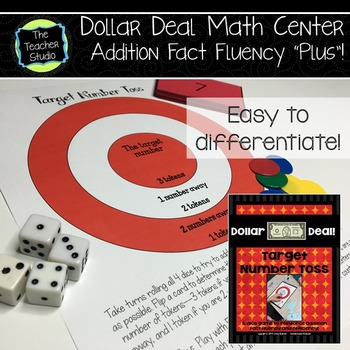# Addition Fact Fluency Game: Dollar Deals: "Target Number"2nd - 4th
Subjects
Standards
Resource Type
Formats Included
• PDF
Pages
5 pages

#### Also included in

1. For teachers using math workshop, guided math, math centers, or are looking to find other ways to differentiate instruction, quality instructional activities are essential. My "Dollar Deal" math games have been a fantastic way for teachers to do just that--at a great price.One thing I have been ask
\$3.50
\$4.00
Save \$0.50
2. Congratulations! You are well on your way to using math games to work more fun into your math block! Math games are one fantastic way to engage students AND teach math concepts. Want a little more background about using games in your math class? CLICKHERE to watch a short video with some ideas and
\$18.00
\$27.00
Save \$9.00

### Description

This resource is an addition fact fluency geared toward helping students add numbers efficiently and to use basic estimation skills. The game requires a game board, the included “target number” cards, and 4 dice. The purpose of this game is to get students to realize that you can add numbers in any order (the associative and commutative properties) and that they can make mental math easier by being strategic about which two numbers to add first. It also works to help students anchor to 10 and develop number sense.

Students roll the dice and use as many of the 4 dice as they need to get as close to the target number as possible. The closer they are, the more “tokens” they earn! This is one of my students’ FAVORITE games, and I have watched them become so much more fluent after playing it for some time!

------------------------------------------------------------------------------

Looking for MORE help teaching addition facts?

BUNDLE of FOUR Games Stressing Addition to 20

------------------------------------------------------------------------------

All rights reserved by ©The Teacher Studio. Purchase of this resource entitles the purchaser the right to reproduce the pages in limited quantities for single classroom use only. Duplication for an entire school, an entire school system, or commercial purposes is strictly forbidden without written permission from the author at fourthgradestudio@gmail.com. Additional licenses are available at a reduced price.

Total Pages
5 pages
N/A
Teaching Duration
N/A
Report this Resource to TpT
Reported resources will be reviewed by our team. Report this resource to let us know if this resource violates TpT’s content guidelines.

### Standards

to see state-specific standards (only available in the US).
Fluently add and subtract within 20 using mental strategies. By end of Grade 2, know from memory all sums of two one-digit numbers.
Add and subtract within 20, demonstrating fluency for addition and subtraction within 10. Use strategies such as counting on; making ten (e.g., 8 + 6 = 8 + 2 + 4 = 10 + 4 = 14); decomposing a number leading to a ten (e.g., 13 - 4 = 13 - 3 - 1 = 10 - 1 = 9); using the relationship between addition and subtraction (e.g., knowing that 8 + 4 = 12, one knows 12 - 8 = 4); and creating equivalent but easier or known sums (e.g., adding 6 + 7 by creating the known equivalent 6 + 6 + 1 = 12 + 1 = 13).
Relate counting to addition and subtraction (e.g., by counting on 2 to add 2).
Understand subtraction as an unknown-addend problem. For example, subtract 10 – 8 by finding the number that makes 10 when added to 8.
Apply properties of operations as strategies to add and subtract. If 8 + 3 = 11 is known, then 3 + 8 = 11 is also known. (Commutative property of addition.) To add 2 + 6 + 4, the second two numbers can be added to make a ten, so 2 + 6 + 4 = 2 + 10 = 12. (Associative property of addition.)